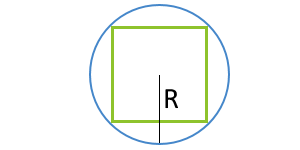# Area of a square calculator

Area of a square calculator - formulas and calculator for calculating square area. Area of a square - is a numerical characteristic characterizing the size of a plane bounded by four series-connected segments (sides) of the same length, whose opposite sides are parallel in pairs, and the angle between any two adjacent sides is 90 degrees.

Different formulas are used to calculate area of a square, depending on the known input data. Below are formulas and a calculator that will help you calculate area or check the already performed calculations.

Area - is a numerical characteristic characterizing the size of a plane bounded by a closed geometric figure.

Area is measured in units of measurement squared: km2, m2, cm2, mm2, etc.

1

## Area of a square through its partya - side

... preparation ...

2

## Area of a square through its diagonal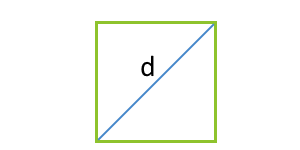d - diagonal

... preparation ...

3

## Area of a square through perimeter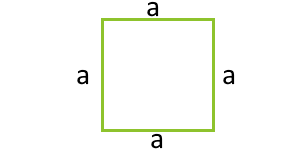P - perimeter
... preparation ...

4

## Area of a square through the piece which is carried out from square top to the middle of the opposite side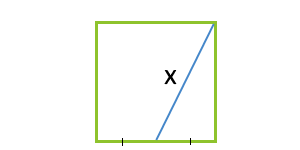k - piece

... preparation ...

5

## Area of a square through an inradius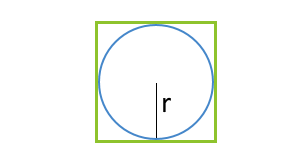r - radius of inscribed circle

... preparation ...

6

## Area of a square through a circumradius